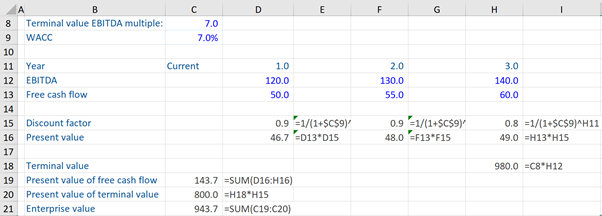## What is a “DCF Terminal Value Formula”?

The process of undertaking DCF analysis (i.e. arriving at a DCF valuation) involves certain steps which include the calculation of the DCF Terminal Value. This can be calculated using two formulas:  the Growing Perpetuity Formula and Terminal EV Multiple Formula.

To understand the concept of DCF Terminal Value, we must know that after a period of growth, a company reaches “steady state” which is when all sources of competitive advantage are exhausted and its profitability and efficiency ratios are stabilized. The steady state period typically coincides with the end of the explicit forecast of the DCF analysis. The value of the future steady state cash flows can be summarized in a single number called the DCF terminal value.

The steps for undertaking DCF analysis are:

1. Forecast the free cash flows to be discounted for the first five or ten years to steady-state (i.e. until the steady-state period)
2. Calculate the weighted average cost of capital (WACC)
3. Compute the terminal value – assume that the company enters into some kind of steady-state – we need to calculate this terminal value and it will represent all those cash flows from year 6 or 11 onwards to infinity
4. Discount the cash flows to today
5. Once all of those future cash flows have been discounted that gives us the enterprise value
6. Finally, calculate the implied share price using the enterprise value to equity bridge.

## Key Learning Points

• The term DCF terminal value summarizes in a single number the value of the future steady-state cash flows
• There are two DCF terminal value formulas that can be used for the calculations – the Growing Perpetuity and Terminal EV Multiple

## DCF Terminal Value Formulas: Growing Perpetuity and Terminal EV Multiple

The DCF Terminal Value is calculated using:

1. Growing Perpetuity Formula:

Terminal Value (TVn)  = Free Cash Flow (FCF)n * (1+g)/(w-g)

w = WACC (weighted average cost of capital)

g = the long-term growth in cash flows.

The terminal value in year n (for example, year 5) equals the free cash flow from year 5 times 1 plus the growth rate (this is really the free cash flow in year 6) divided by the WACC (w) – growth rate (g).

1. Terminal EV Multiple Formula:

Terminal Value (TVn) = LTM EBITDAn * Multiple

N = year 5

The terminal value in year 5 is going to be the last 12 months EBITDA to the end of year 5 multiplied by some kind of ratio. As we are multiplying it by EBITDA, the ratio multiple is going to be EV/EBITDA. This ratio can be a sector or peer group multiple derived from outside the company. It is usually similar companies that have already reached the steady-state and are in their terminal value period. So we take this EV/EBITDA and put it into this formula above.

## DCF Terminal Value Formula, An Example

Given below is a simple DCF workout, where we calculate the DCF Terminal Value (using Terminal EV Multiple Formula) and the Enterprise Value for this company, assuming that cash flows fall at the end of the year.We have a Terminal Value EBITDA multiple of 7.0x. So, in order to calculate the Terminal Value, we have to find the EBITDA multiplied by 7. Then we have a WACC of 7% – which we use for discounting. Thereafter, we have the years, EBITDA, and Free Cash Flows.

So, first we calculate the discount factor, which is equal to 1/(1+WACC of 7%) to the power of the year we are in. This formula gives us the discount factor for each year. Then we calculate the Present Value for each of the years – which is equal to free cash flow multiplied by the discount factor for each year. So, we get present values for years 1, 2, and 3.

Next, we compute the DCF terminal value. The terminal value is calculated by taking the multiple of 7.0x  (refer to column C8) and multiplying it by the EBITDA in year 3 (in this case 140, which is the last year of the detailed cash flows). This calculation gives us a terminal value of 980.0 (shown in cell H18). Then we compute the present value of cash flows which comes to 143.7 (i.e. adding the present value of free cash flows of years 1, 2, and 3).

Thereafter, we can calculate the present value of the terminal value i.e. we take the value of 980 and multiply it by the year 3 discounting factor (which is 0.8). Finally, the Enterprise Value (943.7) is obtained by adding the present value of free cash flows of years 1, 2, and 3 and the present value of terminal value – representing years 4 and onwards.